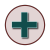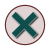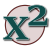Games
Problems
Go Pro!

# Expressions

Lesson Plans > Mathematics > Algebra > Expressions

# Featured Lesson PlansConverting English language phrases to algebraic expressionsAlgebraic expressions - practicing converting more complex phrases into algebraic expressionsAlgebraic expressions worksheets involving two variables, and a substitutionConverting English language phrases into algebraic expressions and arithmetic expressionsA series of worksheets for students on squaring binomialsAlgebraic expressions worksheets in which students must convert phrases to expressions in multiple waysStudents often make mistakes in writing algebraic expressions; the following technique can helpUnderstanding operations, relationships and grouping symbols, as a precursor to building arithmetic expressions and algebraic expressions

# Full Directory Listing

Algebraic Expressions and English Phrases, Algebraic Expressions Worksheets 1, Algebraic Expressions Worksheets 2, Algebraic Expressions Worksheets 3, Algebraic Expressions Worksheets 4, Algebraic Properties Don't Change Anything, Expressions, Operations, Grouping Symbols, and Relationships, Rewriting the Substitution Property, Squaring Binomials, Testing Your Algebraic ExpressionsLike us on Facebook to get updates about new resources